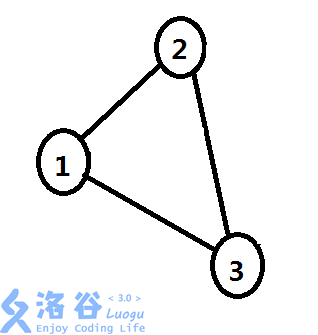# P4892 GodFly的寻宝之旅

• 73通过
• 240提交
• 题目提供者 Forward_Star
• 评测方式 云端评测
• 标签 位运算,按位 枚举,暴力 状态压缩,状压
• 难度 省选/NOI-
• 时空限制 3000ms / 512MB
• 提示：收藏到任务计划后，可在首页查看。

## 题目背景

“蒹葭苍苍，白露为霜。所谓伊人，在水一方…”

怀着$a$ $burning$ $desire$，$GodFly$开启了他追寻学妹之路。

## 题目描述

我们把校园抽象成一个具有$n$个点的无向连通图，其中的$n$个结点分别编号为$1,2,3,...,n$。把$GodFly$经过的结点表示为一个路径集合$A=\left\{a_1,a_2,a_3,...,a_m\right\}$，表示他依次经过了编号为$a_1$、$a_2$、…、$a_m$的结点，由于集合的元素具有互异性，这意味着$GodFly$无法重复经过同一个结点。

$GodFly$现在要从第$1$个结点走到第$n$个结点，然而他的腿疾对他造成了许多不便。定义$GodFly$经过了$m$个结点，当前在点$a_m$，且路径集合$A=\left\{a_1,a_2,a_3...,a_{m-1}\right\}$（加入新结点$a_m$前）时，他的总体力耗费为$w_m=(w_{m-1}+a_m*sum(A))$%$2$，其中$w_{m-1}$表示上一个路径集合的体力耗费；且对于集合$A$，$sum(A)=a_1+a_2+...+a_{m-1}$。

对于$w=0$的情况，我们称$GodFly$处于“滑基态”，否则对于$w=1$的情况，我们称$GodFly$处于“对偶态”。现在$GodFly$想要知道，他走到$n$结点后处于滑基态或对偶态的方案数，由于这个数可能很大，你只需要输出它对$19260817$取膜（模）的结果；注意两个方案是不同的，当且仅当它们有至少一条经过的边不同，而非路径集合不同。

注意：T3压缩包内第一个数据有误，以题面的样例为准。

## 输入输出格式

输入格式：

第一行，两个数$n$，$k$，分别表示点数和边数；

接下来$k$行，每行两个数$u$、$v$，表示结点$u$与结点$v$之间有一条边。

输入的最后一行为一个数$c$，$c=0$表示求滑基态方案数，$c=1$表示求对偶态方案数。

输出格式：

一行，一个数表示方案数，详见题面。

## 输入输出样例

输入样例#1： 复制
3 3
1 2
2 3
1 3
1
输出样例#1： 复制
2

## 说明

【数据范围】

对于$30$%的数据，$n<=10$，$k<=45$，无重边及自环；

对于$60$%的数据，$n<=15$，$k<=300$；

对于$80$%的数据，$n<=15$，$k<=100000$；

对于$100$%的数据，$n<=18$，$k<=100000$；

样例数据在data.zip\fantasy\中。

【样例说明】如图，初始时在$1$结点，路径集合为$\left\{1\right\}$，费用为$0$；

若从$1$走到$2$结点再走到$3$结点，到$2$结点时，费用为$(0+2*sum(\left\{1\right\}))$%$2=2*1$%$2=0$，并把$2$加入路径集合，则此时路径集合为$\left\{1,2\right\}$；到$3$结点时，因上一次费用为0，费用为$(0+3*sum(\left\{1,2\right\}))$%$2=3*(1+2)$%$2=1$；

若从$1$结点直接走到$3$结点，则费用为$(0+3*sum(\left\{1\right\}))$%$2=3*1$%$2=1$。

故最终走到$3$结点时费用为$1$的方案数为$2$。

【提示】

本题时限$3s$，且可以开启$O_2$优化，不必过分担心卡常数，但请确保算法足够优美。

提示
标程仅供做题后或实在无思路时参考。
请自觉、自律地使用该功能并请对自己的学习负责。
如果发现恶意抄袭标程，将按照I类违反进行处理。